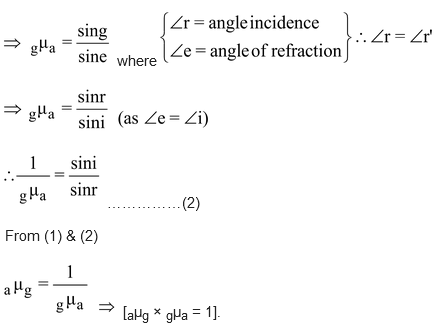# Optical Density

## Light of Class 10

### OPTICAL DENSITY

In the comparison of two media, the medium, or substance having higher refractive index or in which the speed of light is less is called an optically denser medium. On the other hand, a medium (or substance) having lower refractive index or in which the speed of light is higher is called an optically rarer medium.

For example, between the two media, glass and water, glass having a higher refractive index is an optically denser medium than water which has a lower refractive index and hence is an optically rarer medium.Higher the refractive index of a medium, lower is the speed of light in that medium and vice-versa.

### OPTICAL DENSITY VS. MASS DENSITY:

Optical density of a substance is different from its mass density. A medium (substance) may have higher optical density than another medium (substance) but its mass density may be lesser. For example oils (Kerosene, Mustard etc.) having a higher refractive index has higher optical density than water which has a lower refractive index, but the mass density of oils (Kerosene, Mustard etc.) is less than that of water, that is why the oils generally float on the water surface.

### THE DIRECTION OF BENDING OF LIGHT:

The two rules which give the direction of bending of a ray of light when it goes from one medium to another are as follows :

• When a ray of light goes from a rarer medium to a denser medium, it bends towards the normal at the interface of the two media.
•  When a ray of light goes from a denser medium to a rarer medium, it bends away from the normal at the interface of the two media.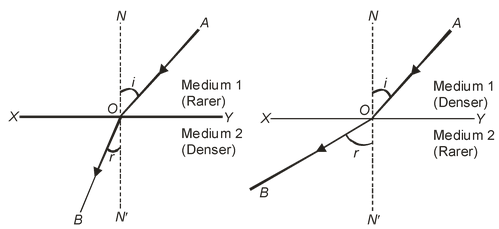Refractive Index in terms of apparent depth and real depth:

Whenever we observe the bottom of a swimming pool or a tank of clear water, we find that the bottom appears to be raised i.e., the apparent depth is less as compared to its real depth.

The extent to which the bottom appears to be raised depends upon the value of refractive index of the refracting medium.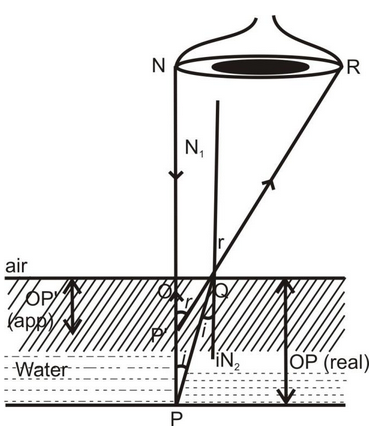In above fig., ∠PQN2 = ∠i & ∠N1OR = ∠rGiven ∠I is very small, it is nearly normal direction of viewing.

PQ ≅ PO

& P’Q ≅ P’O

from (4)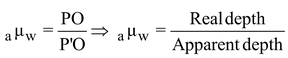.

Refraction and Speed of Light:

The refraction of light occurs because light has different speed in different media. Speed of light is maximum in vacuum or air. It is less in any other medium. Denser in the medium lesser is the speed of light. Refractive index of a medium depends not only on its nature and physical conditions, but also on the colour or wavelength of light. It is more for violet light and less for red light (VIBGYOR).

To find refractive index of two media w.r.t. each other when their refractive indices w.r.t. air are given. A ray of light AB refracts from different medium as shown in figure below.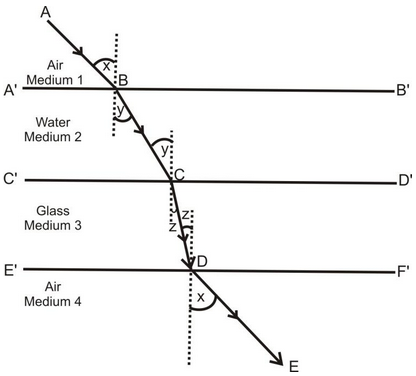(i) For refractive index at interface A’B’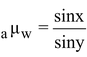..................(i)

(ii) For refractive index at interface C’D’..................(ii)

(iii) For refractive index at interface E’F’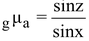.................(iii)

Multiply (1), (2) & (3)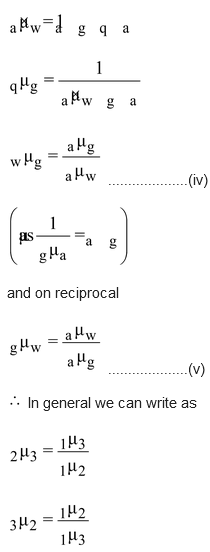Ex. Calculate the speed and wavelength of light (i) in glass & (ii) in air, when light waves of frequency 6 × 1014 Hz. travel from air to glass of μ = 1.5.

Solution: Here v = 6 × 1014 Hz. μ = 1.5

(i) In glass speed of light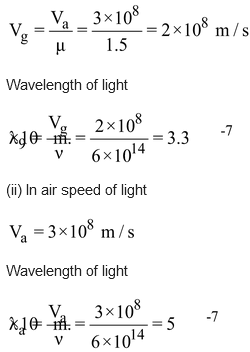### REFRACTION THROUGH A GLASS SLAB(considering no absorption):

Refraction through a rectangular glass slab:

Consider a rectangular glass slab, as shown in the figure. A ray AE is incident on the face PQ at an angle of incidence I. On entering the glass slab, it bends towards normal and travels along EF at an angle of refraction r. The refracted ray EF is incident on face SR at an angle of incidence r’. The emergent ray FD bends away from the normal at an angle of refraction e.

Thus the emergent ray FD is parallel to the incident ray AR, but it has been laterally displaced with respect to the incident ray. There is shift in the path of light on emerging from a refracting medium with parallel faces.

Lateral shift:

Lateral shift is the perpendicular distance between the incident and emergent rays when light is incident obliquely on a refracting slab with parallel faces.

Factors on which lateral shift depends are :

• Lateral shift is directly proportional to the thickness of glass slab.
•  Lateral shift is directly proportional to the incident angle.
•  Lateral shift is directly proportional to the refractive index of glass slab.
• Lateral shift is inversely proportional to the wavelength of incident light.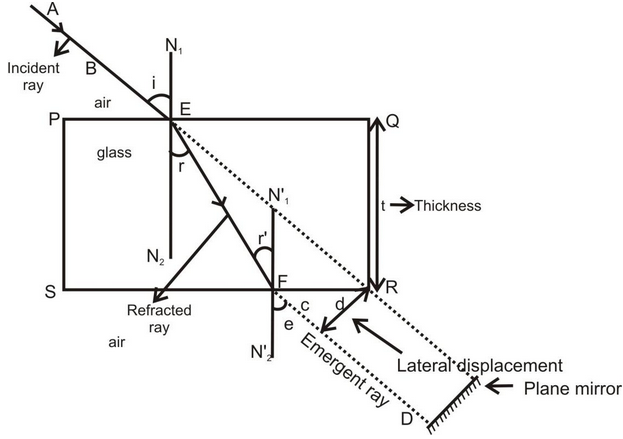Lateral shifting of light in glass slab

If a plane mirror is placed in the path of emergent ray FD then the path of the emergent ray along FD is reversed back, it follows the same path along which it was incident i.e. the incidence ray becomes the emergent ray & emergent ray becomes the incident ray. It is known as principle of reversibility of light.

Case - I : For light going from air to glass of point E.

∠i = angle of incident, ∠r angle of refraction.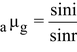.................(1)

( aμg= absolute refractive index of glass)

Case - II : For light going from glass to air at point F.